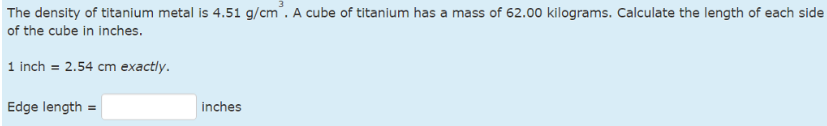# Problem: The density of titanium metal is 4.51 g/cm3. A cube of titanium has a mass of 62.00 kilograms. Calculate the length of each side of the cube in inches. 1 inch = 2.54 cm exactly.

###### FREE Expert Solution
98% (369 ratings)###### Problem Details

The density of titanium metal is 4.51 g/cm3. A cube of titanium has a mass of 62.00 kilograms. Calculate the length of each side of the cube in inches.

1 inch = 2.54 cm exactly.